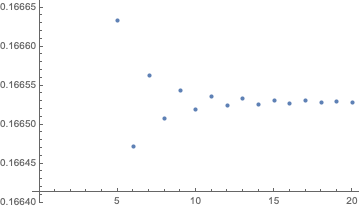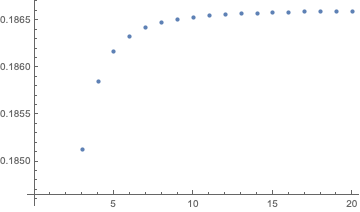# On double series involving Gregory coefficients and quotients of particular values of the gamma function

Edited, there was a mistake. I've edited because there was a mistake, advertised from the answerer, I hope that now all is right.

Yesterday I got (but I haven't tested it numerically) that $$\frac{\sqrt{\pi}}{2}\sum_{n=1}^\infty \sum_{k=1}^\infty\frac{|G_n|}{k}\frac{\Gamma(n+k+\frac{1}{2})}{(n+k+1)!}=\frac{\pi(-5+8\log 2)}{8},$$ where $$G_n$$ denotes the sequence of Gregory coefficients or reciprocal logarithmic numbers, see the Wikipedia Gregory coefficients. Using the generating function for this sequence and the result of the Problem 4383 from Crux Mathematicorum (), I can then write the closed-form of a series of the type

$$\sum_{n=1}^\infty \sum_{k=1}^\infty(\text{a function of }G_n\text{, or }|G_n|,\text{ and }k)\cdot(\text{particular values of the gamma function}).$$

Question. Are known series of previous type and how are evaluated? If it is in the literature refer it, and I try to search and read those statements from the literature. In other case, what work can be done to deduce similar formulas to get the closed-form for double series of the previous type? Many thanks.

Here I add my example of series, how I got the result for the series of the first paragraph.

Example. All issues of convergence are satisfied by uniform convergence of the series in the unit interval. Multiplying the Maclaurin series of the Gregory coefficients, specialized for $$1-x$$, by $$\sqrt{\frac{1-x}{x}}$$ and integrating the equation over the unit interval, one gets invoking the Problem 4383, due to Michel Bataille, that $$-\sum_{n=1}^\infty |G_n|\left(\int_0^1(\log (1-x))\sqrt{\frac{1-x}{x}}x^n dx\right)=\frac{\pi(-5+8\log 2)}{8}.\tag{1}$$ We make a change of varialbe use the Taylor series of the logarithm to state that $$\sum_{n=1}^\infty \sum_{k=1}^\infty\frac{|G_n|}{k}\left(\int_0^1 z^{n+k} \sqrt{\frac{1-z}{z}}dz\right)=\frac{\pi(-5+8\log 2)}{8},\tag{2}$$ and finally I've used Wolfram Alpha online calculator (it is the definition of the gamma function) to get a closed-form of the integral in terms of particular values of the gamma function.

## References:

 Problem 4383, Crux Mathematicorum, Volume 45, Number 6, July 2019.

• The web page of Crux Mathematicorum (I believe that is a journal of the Canadian Mathematical Society) is cms.math.ca/crux from which you can see its Digital Archive. Sep 4, 2019 at 5:45

The result in the OP

$$\sum_{n=1}^\infty \sum_{k=1}^\infty\frac{G_n}{k}\frac{\Gamma(k+\frac{3}{2})\Gamma(n+\frac{1}{2})}{(n+k+1)!}=\frac{\pi(1-8\log 2)}{8}=-1.78489,$$ does not seem to agree with a numerical estimate of the series. I first evaluated the sum over $$k$$, which has a closed form expression, $$\sigma_n=\sum _{k=1}^{\infty } \frac{\Gamma \left(k+\frac{3}{2}\right) \Gamma \left(n+\frac{1}{2}\right)}{k (k+n+1)!}=\frac{\sqrt{\pi }\, \Gamma \left(n+\frac{1}{2}\right) \left(\psi ^{(0)}(n+2)-\psi ^{(0)}\left(n+\frac{1}{2}\right)\right)}{2 \Gamma (n+2)},$$ with $$\psi^{(0)}$$ the polygamma function, and then evaluated $$S_N=\sum_{n=1}^N G_n \sigma_n,$$ using the following Mathematica code for the Gregory coefficients:

g[n_] := Integrate[x*Pochhammer[x - n + 1, n - 1]/n!, {x, 0, 1}]

The plot below of $$S_N$$ versus $$N$$ (evaluated for $$N$$ up to 20) suggests convergence to 0.16653, far from the result in the OP.new version: the OP has a new series

$$\sum_{n=1}^\infty \sum_{k=1}^\infty\frac{|G_n|}{k}\frac{\Gamma(k+\frac{3}{2})\Gamma(n+\frac{1}{2})}{(n+k+1)!}=\frac{\pi(-5+8\log 2)}{8}=0.214091,$$

which I have also evaluated numerically and seems to converge to 0.1866.• Many thnanks I am going to check my calculations in next few hours. Sep 4, 2019 at 8:38
• I've added a new expression, many thanks and I'm sorry. Sep 4, 2019 at 9:41
• My new expression involves the absolute value of the Gregory coefficients, that is $|G_n|$, thus I don't know if my second proposal is true or false. Sep 4, 2019 at 11:05
• This is my new expression, I hope that now it is right. Sep 4, 2019 at 11:55
• Then if possible please delete your answer, with the purpose to delete my own bad post. Many thanks. Sep 4, 2019 at 12:17